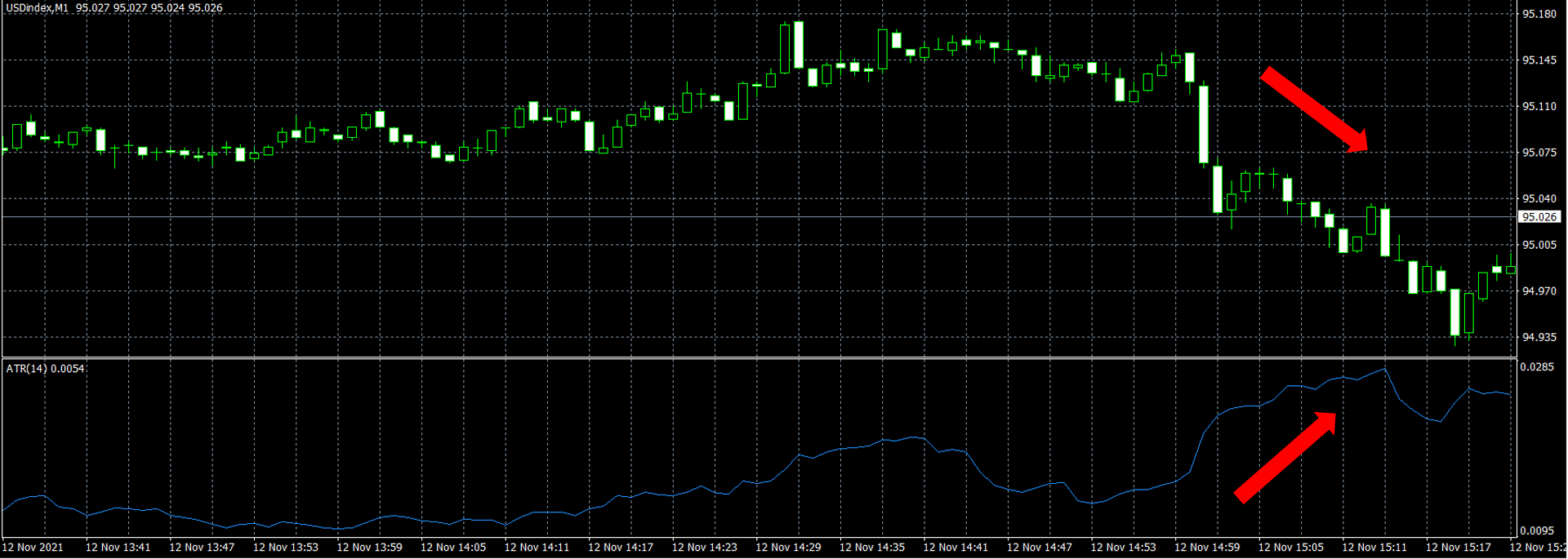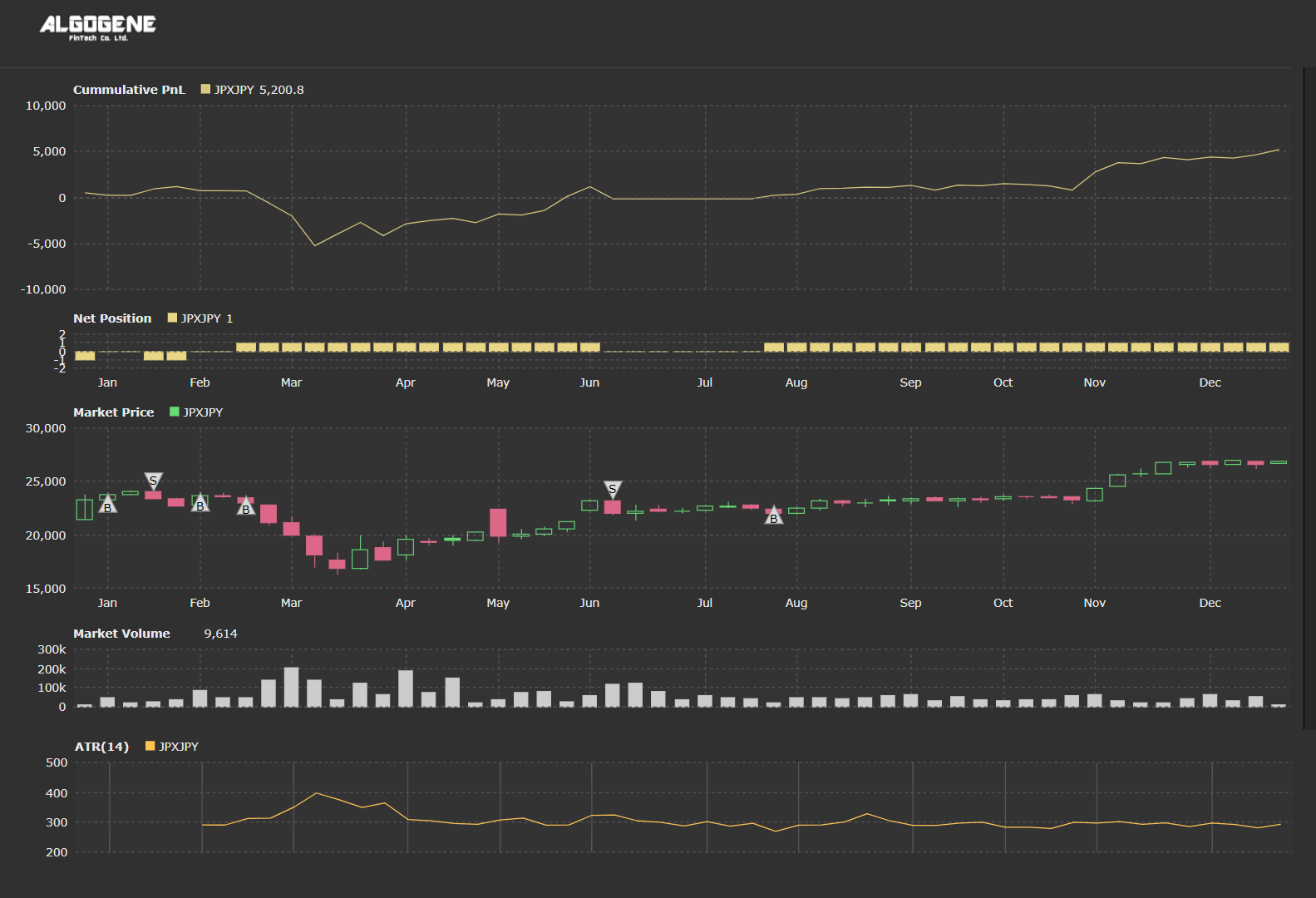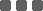## A simple ATR strategy

From the discussion in the previous post, ATR itself does not provide indication about price trend. Instead, it is used to measure market volatility. A stock with a high level of volatility usually has a higher ATR, and similarly, a stock with a lower volatility has a lower ATR.

An old trading saying that "Market take the stairs up and the elevator down." This phenomenon is explained by the fear factor from Behavioral Finance, which is the way how the equity market has been functioning for centuries. As seen from the chart below, during downtrends, the ATR indicator tends to post higher volatility than during up trend.Thus, we can use ATR as a stop loss indicator for order exit and to protect downside risk (so called Chandelier Exit). The chandelier exit places a trailing stop under the highest high the stock reached since you entered the trade. The distance between the highest high and the stop level is defined as some multiple of the ATR. For example,

• if you buy a stock at \$100 and the ATR value is 3, you may place a stop loss at 2 x ATR below the entry price. i.e. 100-2*3 = \$94,
• if you short-sell a stock at \$100 and the ATR is 3, you may place a stop loss at 2 x ATR above the entry price. i.e. 100+2*3 = \$106
• when the market price continues to rise (drop for a sell order), we will revise the stop loss level upward (downward) with the newly calculated ATR multiple

Suppose we use RSI(14) for order entry (refer to Technical Indicator - Relative Strength Index (RSI)) and ATR(14) for order exit, and apply this strategy to the daily closing price of Nikkei 225 Index CFD (i.e. JPXJPY) over the year of 2020.

• Based on a sliding window approach to collect the previous 14 closing price
• Calculate the latest ATR and RSI values
• Order open conditions:
• if we have NO outstanding position,
• if RSI value < 30, we open a buy order with stoploss level set to current price - 2*ATR
• if RSI value > 70, we open a sell order with stoploss level set to current price + 2*ATR
• Order close conditions:
• if we have outstanding position,
• if we previously submit a buy order, update the stop-loss level to the maximum of the previous stop-loss and (current price - 2*ATR)
• if we previously submit a sell order, update the stop-loss level to the minimum of the previous stop-loss and (current price + 2*ATR)
• Repeat the process until the backtest period end

Now, let's write down our trading algorithm step-by-step.

### Step 1. Calculate RSI and ATR value

First of all, we define 'RSI_period', 'ATR_period' and 'instrument' at initialization.

 ``` 1 2 3 4 5 6 7 8 9 10 11``` ```from AlgoAPI import AlgoAPIUtil, AlgoAPI_Backtest from datetime import datetime, timedelta from talib import RSI, ATR import numpy as np class AlgoEvent: def __init__(self): self.timer = datetime(1970,1,1) self.RSI_period = 14 self.ATR_period = 14 self.instrument = "JPXJPY" ```

We use the API function getHistoricalBar to collect historical observations. Then, we apply a python library 'talib.RSI', 'talib.ATR' to calculate the latest RSI and ATR value.

 ``` 1 2 3 4 5 6 7 8 9 10 11 12 13 14 15 16 17 18 19 20``` ``` def on_marketdatafeed(self, md, ab): # execute stratey every 24 hours if md.timestamp >= self.timer+timedelta(hours=24): # get last 14 closing price res = self.evt.getHistoricalBar({"instrument":self.instrument}, self.RSI_period+1, "D") arrClose = np.array([res[t]['c'] for t in res]) arrHigh = np.array([res[t]['h'] for t in res]) arrLow = np.array([res[t]['l'] for t in res]) # calculate the current RSI value RSI_cur = RSI(arrClose, self.RSI_period)[-1] # calculate the current ATR value ATR_cur = ATR(arrHigh, arrLow, arrClose, self.ATR_period)[-1] # print out RSI and ATR value to console self.evt.consoleLog("RSI_cur, ATR_cur = ", RSI_cur, ATR_cur) # update timer self.timer = md.timestamp ```

### Step 2. Order Entry Conditions

We further define 'rsi_overbought', 'rsi_oversold' and 'ATR_multiple' at initialization.

 ``` 1 2 3 4 5 6 7 8 9 10 11 12 13 14 15``` ```from AlgoAPI import AlgoAPIUtil, AlgoAPI_Backtest from datetime import datetime, timedelta from talib import RSI, ATR import numpy as np class AlgoEvent: def __init__(self): self.timer = datetime(1970,1,1) self.timer = datetime(1970,1,1) self.RSI_period = 14 self.ATR_period = 14 self.instrument = "JPXJPY" self.rsi_overbought = 70 self.rsi_oversold = 30 self.ATR_multiple = 2 ```

We also create a function 'open_order' to handle order submissions.

 ``` 1 2 3 4 5 6 7 8 9 10 11 12 13 14 15 16 17 18 19 20 21 22``` ``` def open_order(self, buysell, current_price, ATR): # set stop loss level to be 2*ATR below current price for buy order if buysell==1: sl = current_price - self.ATR_multiple*ATR # set stop loss level to be 2*ATR above current price for buy order else: sl = current_price + self.ATR_multiple*ATR # create order object order = AlgoAPIUtil.OrderObject( instrument = self.instrument, openclose = 'open', buysell = buysell, #1=buy, -1=sell ordertype = 0, #0=market, 1=limit volume = 0.01, stopLossLevel = sl ) # send order to server self.evt.sendOrder(order) ```

Now, we update the system's function 'on_marketdatafeed' for order open logic.

 ``` 1 2 3 4 5 6 7 8 9 10 11 12 13 14 15 16 17 18 19 20 21 22 23 24 25 26 27 28 29 30 31 32 33 34 35 36 37``` ``` def on_marketdatafeed(self, md, ab): # execute stratey every 24 hours if md.timestamp >= self.timer+timedelta(hours=24): # get last 14 closing price res = self.evt.getHistoricalBar({"instrument":self.instrument}, self.RSI_period+1, "D") arrClose = np.array([res[t]['c'] for t in res]) arrHigh = np.array([res[t]['h'] for t in res]) arrLow = np.array([res[t]['l'] for t in res]) # calculate the current RSI value RSI_cur = RSI(arrClose, self.RSI_period)[-1] # calculate the current ATR value ATR_cur = ATR(arrHigh, arrLow, arrClose, self.ATR_period)[-1] # print out RSI and ATR value to console self.evt.consoleLog("RSI_cur, ATR_cur = ", RSI_cur, ATR_cur) # get outstanding position pos, osOrder, pendOrder = self.evt.getSystemOrders() # open an order if we have no outstanding position if pos[self.instrument]["netVolume"]==0: # open a sell order if it is overbought if RSI_cur>self.rsi_overbought: self.open_order(-1, md.lastPrice, ATR_cur) # open a buy order if it is oversold elif RSI_cur

### Step 3. Order Close Condition

For order closing logic, we recalculate and update the new stop loss level in 'on_marketdatafeed' function. (refer to line 36 - 49)

 ``` 1 2 3 4 5 6 7 8 9 10 11 12 13 14 15 16 17 18 19 20 21 22 23 24 25 26 27 28 29 30 31 32 33 34 35 36 37 38 39 40 41 42 43 44 45 46 47 48 49 50 51 52 53``` ``` def on_marketdatafeed(self, md, ab): # execute stratey every 24 hours if md.timestamp >= self.timer+timedelta(hours=24): # get last 14 closing price res = self.evt.getHistoricalBar({"instrument":self.instrument}, self.RSI_period+1, "D") arrClose = np.array([res[t]['c'] for t in res]) arrHigh = np.array([res[t]['h'] for t in res]) arrLow = np.array([res[t]['l'] for t in res]) # calculate the current RSI value RSI_cur = RSI(arrClose, self.RSI_period)[-1] # calculate the current ATR value ATR_cur = ATR(arrHigh, arrLow, arrClose, self.ATR_period)[-1] # print out RSI and ATR value to console self.evt.consoleLog("RSI_cur, ATR_cur = ", RSI_cur, ATR_cur) # get outstanding position pos, osOrder, pendOrder = self.evt.getSystemOrders() # open an order if we have no outstanding position if pos[self.instrument]["netVolume"]==0: # open a sell order if it is overbought if RSI_cur>self.rsi_overbought: self.open_order(-1, md.lastPrice, ATR_cur) # open a buy order if it is oversold elif RSI_cur

### Full Source Code

Combining all above, the full script is presented below.

 ``` 1 2 3 4 5 6 7 8 9 10 11 12 13 14 15 16 17 18 19 20 21 22 23 24 25 26 27 28 29 30 31 32 33 34 35 36 37 38 39 40 41 42 43 44 45 46 47 48 49 50 51 52 53 54 55 56 57 58 59 60 61 62 63 64 65 66 67 68 69 70 71 72 73 74 75 76 77 78 79 80 81 82 83 84 85 86 87 88 89 90 91 92 93 94 95 96 97 98 99 100 101 102 103 104 105 106 107 108 109 110 111 112 113 114 115 116 117 118 119 120``` ```from AlgoAPI import AlgoAPIUtil, AlgoAPI_Backtest from datetime import datetime, timedelta from talib import RSI, ATR import numpy as np class AlgoEvent: def __init__(self): self.timer = datetime(1970,1,1) self.timer = datetime(1970,1,1) self.RSI_period = 14 self.ATR_period = 14 self.instrument = "JPXJPY" self.rsi_overbought = 70 self.rsi_oversold = 30 self.ATR_multiple = 2 def start(self, mEvt): self.evt = AlgoAPI_Backtest.AlgoEvtHandler(self, mEvt) self.evt.start() def on_marketdatafeed(self, md, ab): # execute stratey every 24 hours if md.timestamp >= self.timer+timedelta(hours=24): # get last 14 closing price res = self.evt.getHistoricalBar({"instrument":self.instrument}, self.RSI_period+1, "D") arrClose = np.array([res[t]['c'] for t in res]) arrHigh = np.array([res[t]['h'] for t in res]) arrLow = np.array([res[t]['l'] for t in res]) # calculate the current RSI value RSI_cur = RSI(arrClose, self.RSI_period)[-1] # calculate the current ATR value ATR_cur = ATR(arrHigh, arrLow, arrClose, self.ATR_period)[-1] # print out RSI and ATR value to console self.evt.consoleLog("RSI_cur, ATR_cur = ", RSI_cur, ATR_cur) # get outstanding position pos, osOrder, pendOrder = self.evt.getSystemOrders() # open an order if we have no outstanding position if pos[self.instrument]["netVolume"]==0: # open a sell order if it is overbought if RSI_cur>self.rsi_overbought: self.open_order(-1, md.lastPrice, ATR_cur) # open a buy order if it is oversold elif RSI_cur

### Results

Now, we are prepared to backtest this strategy.

Backtest Settings:

• Instrument: JPXJPY
• Period: 2020.01 - 2020.12
• Initial Capital: US\$10,000
• Data Interval: 1-day bar
• Leverage: 100
• Allow Shortsell: True

Backtest Result### Final Thoughts

Some highlights for applying ATR in a trading strategy as below:

• ATR is mainly used to determine order exit condition, but not for order entry
• Multi-period ATRs (eg. 5 and 14) could be used to compare and have a sense about the recent "market volatility"
• The rule of thumb for ATR period is 14
• Is there any better choice?
• Can this value be dynamically changing?
• Will it be generally applicable to other financial instruments/ asset classes?

### Categories

Hot Topic

47 0 2 2022-06-29

138 0 3 2022-06-05

Initial capital is missing in livetest?

329 1 0 2022-05-19

AlgoBot partners with coding lab Algogene for code-to-earn

403 0 3 2022-05-10

A trend following strategy based on volatility approach

216 0 2 2022-05-09

All you need to know about Sharpe Ratio

391 1 2 2022-04-25

447 1 1 2022-04-08

What is the winning rate of a trading strategy?

263 4 0 2022-03-28